+
Solving Mixture Problems using Weighted Average
Solving Linear Equations and Inequalities
0
of 0 possible points

# Solving Mixture Problems using Weighted Average

Author: Sophia Tutorial
##### Description:

Use weighted average to calculate the concentration of a mixed solution.

(more)

Sophia’s self-paced online courses are a great way to save time and money as you earn credits eligible for transfer to many different colleges and universities.*

No credit card required

37 Sophia partners guarantee credit transfer.

299 Institutions have accepted or given pre-approval for credit transfer.

* The American Council on Education's College Credit Recommendation Service (ACE Credit®) has evaluated and recommended college credit for 33 of Sophia’s online courses. Many different colleges and universities consider ACE CREDIT recommendations in determining the applicability to their course and degree programs.

Tutorial
what's covered
1. Average/Mean
2. Weighted Average
3. Mixture Problems

# 1. Average/Mean

Before we begin discussing weighted average, it may be helpful to review how to calculate the simple average or mean of a data set. In order to find the average, we find the sum of all data values, and then divide by the number of data values in our set. This is illustrated in the example below: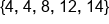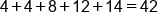Add all values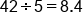Divide sum by number of data values, 5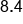Our Solution

# 2. Weighted Average

Weighted average is different from simple average in that certain data values carry more weight, or influence the average more heavily. With simple average, every data point is equally represented in the calculation for the mean, but in weighted averages, some data points are multiplied by a number in its calculation.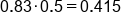Weighted test/project grade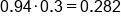Weighted assignment grade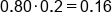Weighted participation grade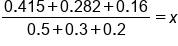Weighted portion in numberator, individual weights in denominator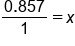Simplify numerator and denominator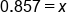Divide 0.857 by 1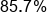Our Solution

In general, data values in the denominator of the fraction are multiplied by its corresponding weight. The corresponding weights are then added together to form the denominator of the fraction. In this case, the weights summed to 100% because the weights of tests/projects, assignments, and participation need to reflect 100% of your grade in total.

# 3. Mixture Problems

Next, we will use the concept of weighted average to set up and solve a mixture problem. Consider the following example:

To prepare for a lab experiment, you mix two concentrations of HCl (hydrochloric acid): 30 mL of 15% HCl solution, and 20 mL of 40% HCl solution. What is the concentration of the mixed solution?

To solve this problem, we need to multiply the quantity of each solution by its concentration (or its weight to be included in our calculation). This will represent the numerator of our fraction for weighted average. As for the denominator, we divide by the total quantity of the solution (so we add the individual amounts to get a total amount of liquid). The solution is worked out below: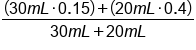Multiply the quantity by its weight in numerator. Add quantities in denominator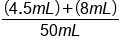Simplify the numerator and denominator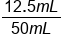Add 4.5 to 8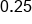Divide by 50mL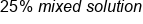Our Solution

Some mixture problems will ask us to find specific amounts of each solution that must be mixed together in order to yield a certain amount of a specific concentration. Let's again use the HCl example. We have two kinds of solutions already made: a 15% solution, and a 40% solution. To prepare for a lab experiment, we are going to need 120 mL of a 33.33% solution. How many mL of each solution must be mixed in order to create this mixture?

Let's define variables for this situation:

• x = mL of 15% solution
• y = mL of 40% solution

We have an immediate relationship between x and y, that x + y = 120. How does this affect our equation for weighted average?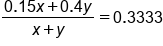Weighted average equation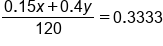x+y=120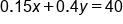Multiply by 120

We have simplified our equation, but it still has two variables. If this equation could be expressed using only 1 variable, we could solve for that variable, and then use substitution again to solve for the other variable. Let's return to the relationship x + y = 120. We can write this equivalently as y = 120 – x.

Now that we have an expression for y, we can write 120 – x into the original equation instead of y. The result will be a single-variable equation, and we'll be able to solve for x.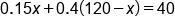y=120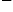x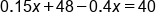Distribute 0.4 into (120x)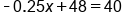Combine like terms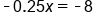Subtract 48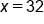Divide by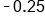This tell us that we must use 32 mL of the 15% solution in our mixture. Since we know we need a total of 120 mL, we can deduce that we'll need 88 mL of the 40% solution. This combination will yield 120 mL of 33.33% solution.

summary
Recall that the average/mean is finding the sum of all data values, and then divide by the number of data values in our set. A weighted average gives you the average of a set of values that may carry different weights. The more weight that a value has, the more it is accounted for in the calculation. We can use the concept of weighted average to calculate mixture problems.

Formulas to Know
Weighted AverageRating